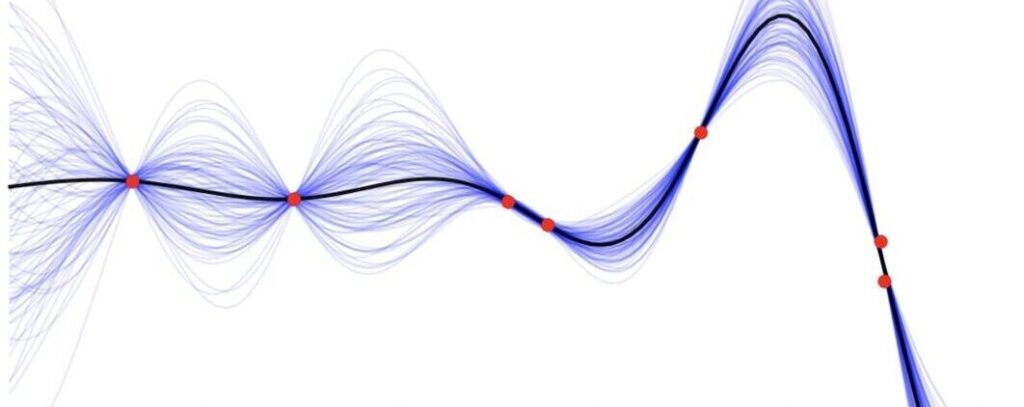1

# 190.013 Introduction to Machine Learning Lab (2SH P, SS)

This course accompanies the 190.012 Introduction to Machine Learning lecture.

Enrolling for this exercise is a highly recommended but not a requirement for passing the machine learning lecture.## Format, Location & Time

• Format: The course format is physical attendance. The lectures are going to be broadcasted via Webex too, but the focus will be given only to participants physically present.
• Location: HS Thermoprozesstechnik
• Dates: Fridays 13:15-15:00

## Lectures

• Assignment 1: Preliminaries and Introduction to Google Collab and Python
• Assignment 2: Programming in Python, Probability Theory & Linear Algebra operations
• python classes
• gaussian distributions
• sampling
• plotting
• Linear Algebra
• Assignment 3: Probability Theory
• Classes for Distributions
• Plotting & Sampling
• Bayes Theorem
• Assignment 4Linear Regression with Diabetes Dataset
• Data Preprocessing
• Least Squares Regression
• Ridge Regression
• Lasso Regression
• Assignment 5: Non-Linear Regression & Classification
• Perceptron
• Non-linear Feature Transformations (Polynomial and RBFs)
• Neural Network
• Assignment 6: Probabilistic Time Series Models ( Gaussian Process)

Details to the grading will be presented in the first exercise on the 03.03.2023. For each assignment code templates will be provided.

## Learning objectives / qualifications

Hands-on experience with machine learning methods.

## Contact

Fotios Lygerakis via email at fotios.lygerakis@unileoben.ac.at